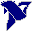# LabVIEW

cancel
Showing results for
Did you mean:

## How to plot a plane by three points with coordinates (x, y, z)

I know the coordinates (x, y, z) of some points and I should plot a plane steps for all points. Someone has already had this problem and know how can I do to solve it?

Message 1 of 4
(1,571 Views)

## Re: How to plot a plane by three points with coordinates (x, y, z)

Hi 12345cor,

LabVIEW comes with several 3D graphs, which accept XYZ data!

Did you try them? Did you look at the example VIs for those graphs?

What exactly do you have problems with?

Best regards,
GerdWusing LV2011SP1 + LV2017 (+LV2020 sometimes) on Win10+cRIO
Message 2 of 4
(1,558 Views)

## Re: How to plot a plane by three points with coordinates (x, y, z)

Hello,

How do you want to plot this data? Using in a map or the path?

The only time I have worked with planes and LabVIEW was to plot the orientation according to IMU information, so I had to use the math transforms for each axis. This is very simple to do.

Can you elaborate more on what do you want to do?

Guilherme CorreaMessage 3 of 4
(1,549 Views)

## Re: How to plot a plane by three points with coordinates (x, y, z)

I need to create a plane that passes through three points of which I know x, y, z. then I must be projected on the plane two segments which intersect this plane and passing through other points of which I know xyz and calculate the angle of inclination between these segments

Message 4 of 4
(1,509 Views)